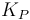# Difference between revisions of "Theory of Operation"

## Formal Methods

The critical core of the Paparazzi code has been proven by formal methods to ensure absolute reliability of the servo, R/C, and manual control portions of the code using the same tools and methods used for full-scale autopilots, nuclear power plants, and other critical control systems. See our page on formally proving critical Paparazzi code using Lustre.

## PID

Paparazzi uses common Proportional Integral Derivative (PID) control for stability and navigation. PID is probably the most commonly used feedback control design as it is simple to implement and intuitive to operate. PID controllers use three terms operating on the measured error to produce a control output. If u(t) is the control signal sent to the system, y(t) is the measured output and r(t) is the desired output, and tracking error$e(t)=r(t)- y(t)$, a PID controller has the general form$u(t) = K_P e(t) + K_I \int e(t)dt + K_D \dot{e}(t)$

The desired closed loop dynamics are obtained by adjusting the three parameters$K_P$,$K_I$ and$K_D$, often iteratively by "tuning" and without specific knowledge of a plant model. Stability can often be ensured using only the proportional term in well damped systems. The integral term compensates for steady long-term errors, and the derivative term is used to provide damping to reduce oscillation.

See more on Wikipedia: PID Controller

Paparazzi uses PID controllers on all loops but many of the I and D terms are not fully implemented as they are often unnecessary or difficult to tune. Below are some of the current PID implementations in Paparazzi.

### Roll Rate

In sw/airborne/fw_h_ctl.c we define the roll rate loop:

float cmd = throttle_dep_pgain * ( err + h_ctl_roll_rate_igain * roll_rate_sum_err / H_CTL_ROLL_RATE_SUM_NB_SAMPLES + h_ctl_roll_rate_dgain * d_err);


the roll Pgain is variable with throttle and multiplies through the entire equation affecting the I and D terms as well for ease of tuning.

### Pitch Angle

In sw/airborne/fw_h_ctl.c we define the pitch angle loop:

 float err = estimator_theta - h_ctl_pitch_setpoint;
float d_err = err - last_err;
last_err = err;
float cmd = h_ctl_pitch_pgain * (err + h_ctl_pitch_dgain * d_err) + h_ctl_elevator_of_roll * fabs(estimator_phi);


Here we use only Proportional as the Derivative is always set to zero. An integral term could prove useful here. Aircraft pitch response is normally very well damped. Those with "plank" style aircraft or other pitch-sensitive designs may benefit from implementing a gyro-based D term.

In sw/airborne/fw_h_ctl.c we define the navigation loop:

 float err = estimator_hspeed_dir - h_ctl_course_setpoint;
float speed_depend_nav = estimator_hspeed_mod/NOMINAL_AIRSPEED;
Bound(speed_depend_nav, 0.66, 1.5);
float cmd = h_ctl_course_pgain * err * speed_depend_nav;


Here we only use the Proportional term though a Derivative would be useful as navigation is not well damped. Note that an Integral term cannot be used for navigtation without accurate and reliable wind information and the necessary implementation of wind data. Note however that we increase/reduce the commanded bank angle for navigation based on the ground speed. This reduces "hunting" on upwind legs, keeps the navigation tight on fast downwind legs and helps keep circles round in a crosswind.

### Climb Rate

In sw/airborne/fw_v_ctl.c we compute the desired climb rate:

v_ctl_altitude_error = estimator_z - v_ctl_altitude_setpoint;
v_ctl_climb_setpoint = v_ctl_altitude_pgain * v_ctl_altitude_error + v_ctl_altitude_pre_climb;


Here we only use the Proportional term though a Derivative would be useful as climb rate is not well damped. An integral term may prove useful if well-implemented. The last term v_ctl_altitude_pre_climb represents the desired constant climb rate needed to follow a 3-dimensional navigation path. This term is zero for level flight, altitude maintenance, and commanded altitude changes.
Then we compute the throttle response:

float err  = estimator_z_dot - v_ctl_climb_setpoint;
float controlled_throttle = v_ctl_auto_throttle_cruise_throttle + v_ctl_auto_throttle_climb_throttle_increment * v_ctl_climb_setpoint
+ v_ctl_auto_throttle_pgain * (err + v_ctl_auto_throttle_igain * v_ctl_auto_throttle_sum_err);


and pitch response

float v_ctl_pitch_of_vz = v_ctl_climb_setpoint * v_ctl_auto_throttle_pitch_of_vz_pgain;